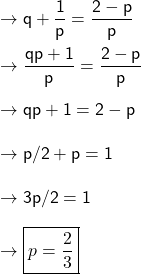## The equation px²+px+3q=1+2x has roots 1/p and q (a) Find the values of p and of q​

Question

The equation px²+px+3q=1+2x has roots 1/p and q

(a) Find the values of p and of q​

in progress 0
6 months 2021-07-23T14:17:04+00:00 1 Answers 25 views 0

p = 2/3

q = 1/2

Step-by-step explanation:

The given equation is ,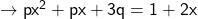We can write it as ,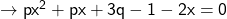Rearrange the terms ,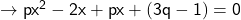This can be written as ,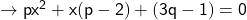Now wrt Standard form of a quadratic equation ,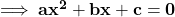we have ,

• a = p
• b = p – 2
• c = 3q – 1

We know that product of zeroes :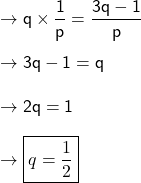Sum of roots :